# Understanding Nested For Loops in JavaScriptself.__wrap_n=self.__wrap_n||(self.CSS&&CSS.supports("text-wrap","balance")?1:2);self.__wrap_b=(e,r,t)=>{t=t||document.querySelector(`[data-br="\${e}"]`);let n=t.parentElement,a=s=>t.style.maxWidth=s+"px";t.style.maxWidth="";let o,i=n.clientWidth,l=n.clientHeight,c=i/2-.25,f=i+.5;if(i){for(a(c),c=Math.max(t.scrollWidth,c);c+1<f;)o=Math.round((c+f)/2),a(o),n.clientHeight===l?f=o:c=o;a(f*r+i*(1-r))}t.__wrap_o||typeof ResizeObserver<"u"&&(t.__wrap_o=new ResizeObserver(()=>{self.__wrap_b(0,+t.dataset.brr,t)})).observe(n)};self.__wrap_n!=1&&self.__wrap_b(":Rj0pm:",1)

November 8, 2023

Nested for loops in JavaScript are a method for iterating over data structures that require multiple levels of looping. They are particularly useful when dealing with multi-dimensional arrays or performing repeated actions within another loop.

## What are nested for loops in JavaScript?

A nested for loop is when you have one for loop inside another for loop. This construction is necessary when you want to perform operations on more complex data structures, like a matrix or a list of lists. Each level of the loop corresponds to one level of depth in the data structure.

## Syntax of a nested for loop

The syntax of a nested for loop follows the standard for loop syntax, but includes another for loop within its code block. Here's an abstract example:

```for (let i = 0; i < outerLimit; i++) {
for (let j = 0; j < innerLimit; j++) {
// Code to execute in the inner loop
}
// Code to execute in the outer loop
}```

## How nested for loops work

The outer loop starts first, and for each iteration of the outer loop, the inner loop runs completely from start to finish. Think of it as a clock where the minute hand (inner loop) completes its cycle before the hour hand (outer loop) moves.

## Use cases for nested for loops

Nested for loops are commonly used in tasks such as:

• Generating multi-dimensional arrays
• Searching elements in a matrix
• Applying functions to grid-like data structures
• Complex sorting algorithms

## Tips for working with nested for loops

• Ensure that inner loops have a different iterator variable.
• Be mindful of the computational cost; nested loops can quickly lead to high time complexity.
• Use descriptive variable names for iterators to keep track of their purpose.

## Example of a nested for loop

Here's an example of a nested for loop that multiplies the elements of two matrices:

```const matrixA = [[1, 2], [3, 4]];
const matrixB = [[5, 6], [7, 8]];
const result = [[0, 0], [0, 0]];

for (let i = 0; i < matrixA.length; i++) {
for (let j = 0; j < matrixB[i].length; j++) {
for (let k = 0; k < matrixA[i].length; k++) {
result[i][j] += matrixA[i][k] * matrixB[k][j];
}
}
}```

This code snippet demonstrates a triple nested for loop, which is used for matrix multiplication. The outermost loop iterates over the rows of `matrixA`, the middle loop iterates over the columns of `matrixB`, and the innermost loop performs the multiplication and sum of elements.

## Handling complexity in nested loops

While nested for loops can be a powerful tool, they can also introduce complexity into your code. Here are some ways to manage this complexity:

• Break down complex operations into functions.
• Consider alternative data structures or algorithms.
• Comment on each level of the loop to explain its role.

## Debugging nested for loops

Debugging nested loops can be challenging due to the multiple levels of iteration. To simplify the debugging process:

• Use `console.log` to track the values of iterator variables.
• Check loop conditions and ensure they are correctly set.
• Look for off-by-one errors, which are common in loops.

Understanding nested for loops is essential for JavaScript developers working with data structures that have more than one dimension. By mastering nested loops, you can manipulate and process complex datasets with ease.

## How to avoid nested for loops in JavaScript

Avoiding nested for loops can lead to more readable and efficient code. Here are some strategies to minimize or eliminate the need for nesting:

### Use higher-order functions

JavaScript arrays come with higher-order functions that can often replace nested loops. Methods like `map()`, `filter()`, `reduce()`, and `forEach()` can perform many of the tasks that you might otherwise use a nested loop for.

### Example with `forEach()`:

```matrixA.forEach((row, i) => {
row.forEach((element, j) => {
// Code that would be inside the nested loop
});
});```

### Flatten data structures

If you're dealing with multi-dimensional arrays, consider whether you can flatten them into a single array. This can often be done when the nested loop is only reading data and not modifying the structure.

### Example of flattening an array:

```const flatArray = matrixA.flat();
flatArray.forEach((element, index) => {
// Code that would be inside the nested loop
});```

### Use recursion

Recursion can be a powerful alternative to nested loops, especially for operations that need to be repeated at multiple depths.

### Example of recursion:

```function processArray(array) {
array.forEach(element => {
if (Array.isArray(element)) {
processArray(element); // Recursive call
} else {
// Process single element
}
});
}```

### Utilize helper functions

Breaking down your nested loops into separate, smaller functions can make your code cleaner and more modular.

### Example of using a helper function:

```function processElement(element) {
// Code to process an individual element
}

matrixA.forEach(row => {
row.forEach(processElement);
});```

### Iterators and Generators

In modern JavaScript, you can use iterators and generators to loop through data in a lazy fashion, which can be more memory-efficient than nested loops and can also lead to cleaner code.

### Example of a generator function:

```function* matrixIterator(matrix) {
for (let row of matrix) {
for (let element of row) {
yield element;
}
}
}

const iterator = matrixIterator(matrixA);
let result = iterator.next();
while (!result.done) {
// Process result.value
result = iterator.next();
}```

# Ship faster, worry less with Basedash

You're busy enough with product work to be weighed down building, maintaining, scoping and developing internal apps and admin panels. Forget all of that, and give your team the admin panel that you don't have to build. Launch in less time than it takes to run a standup.

You're busy enough with product work to be weighed down building, maintaining, scoping and developing internal apps and admin panels. Forget all of that, and give your team the admin panel that you don't have to build. Launch in less time than it takes to run a standup.

You're busy enough with product work to be weighed down building, maintaining, scoping and developing internal apps and admin panels. Forget all of that, and give your team the admin panel that you don't have to build. Launch in less time than it takes to run a standup.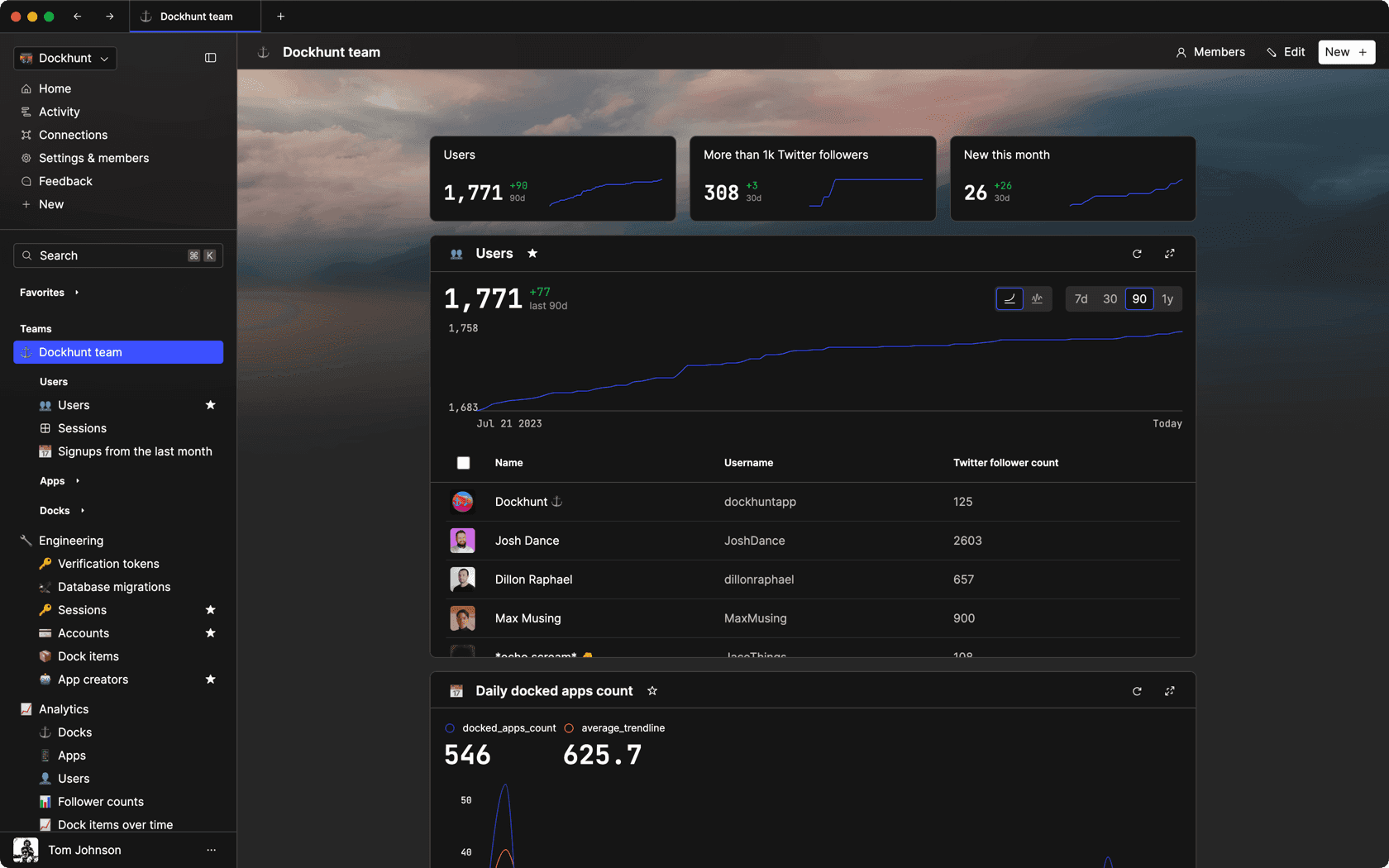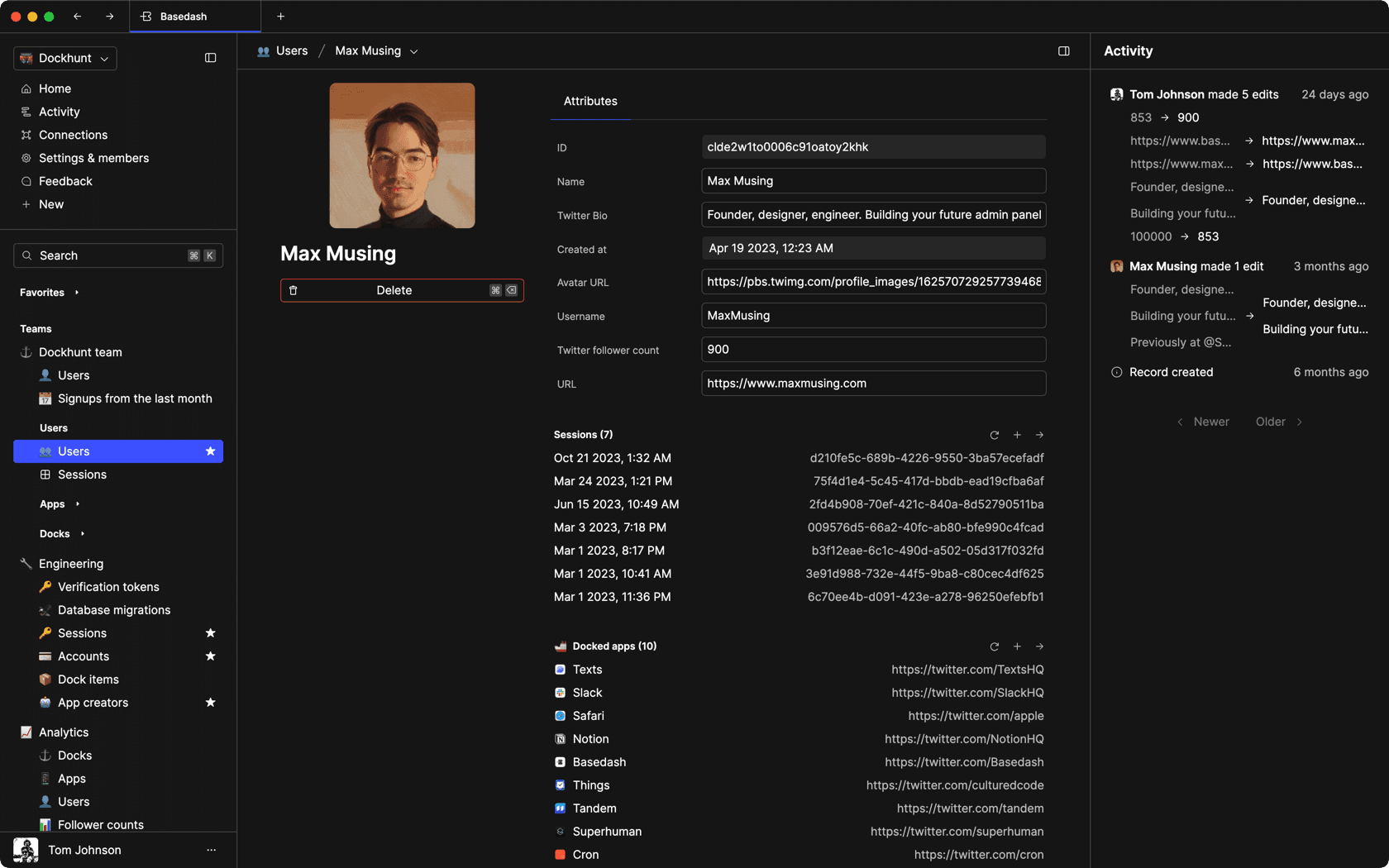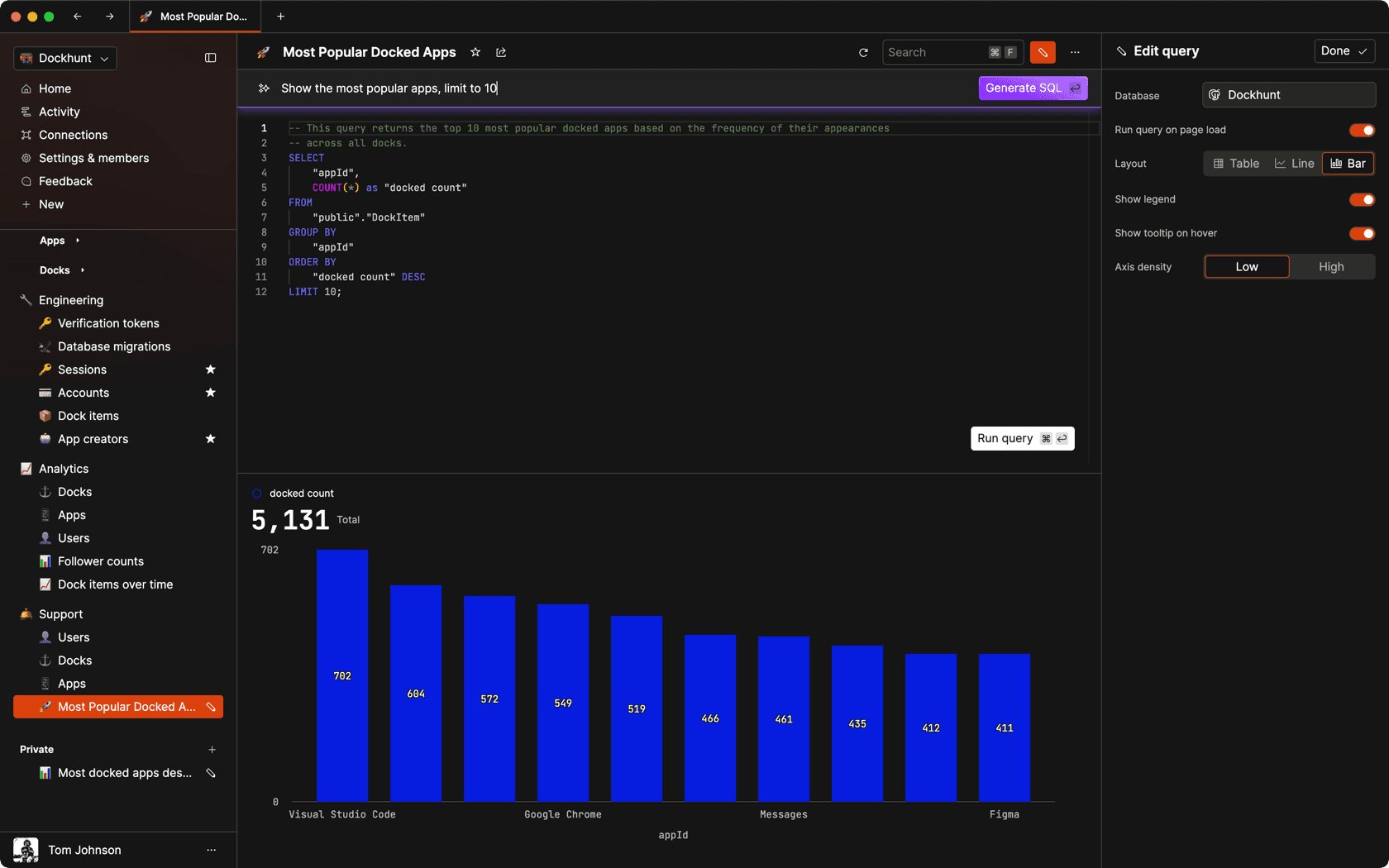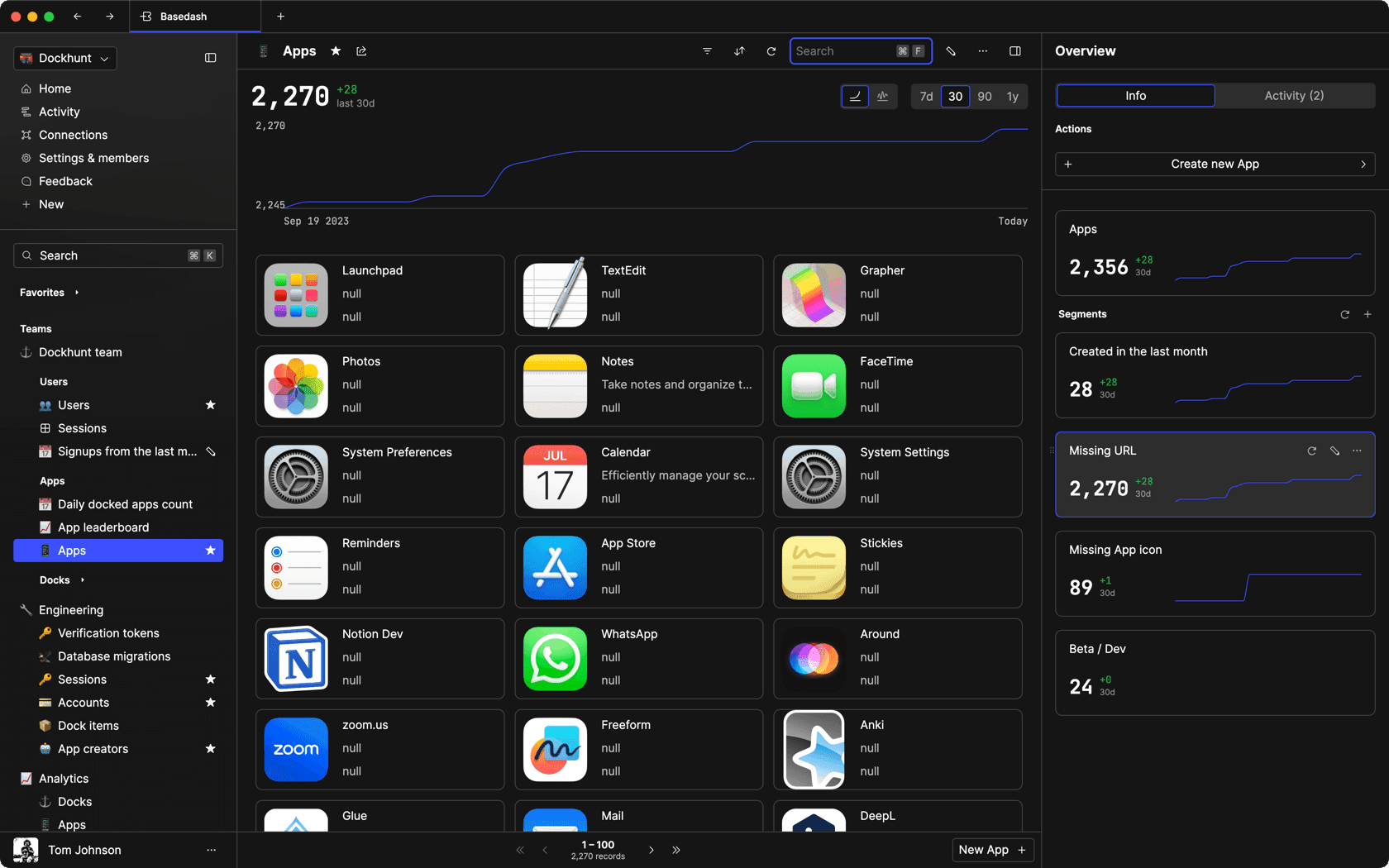### Dashboards and charts

Effortlessly make charts and create a space for your team to work together towards shared goals and metrics.

### SQL composer with AI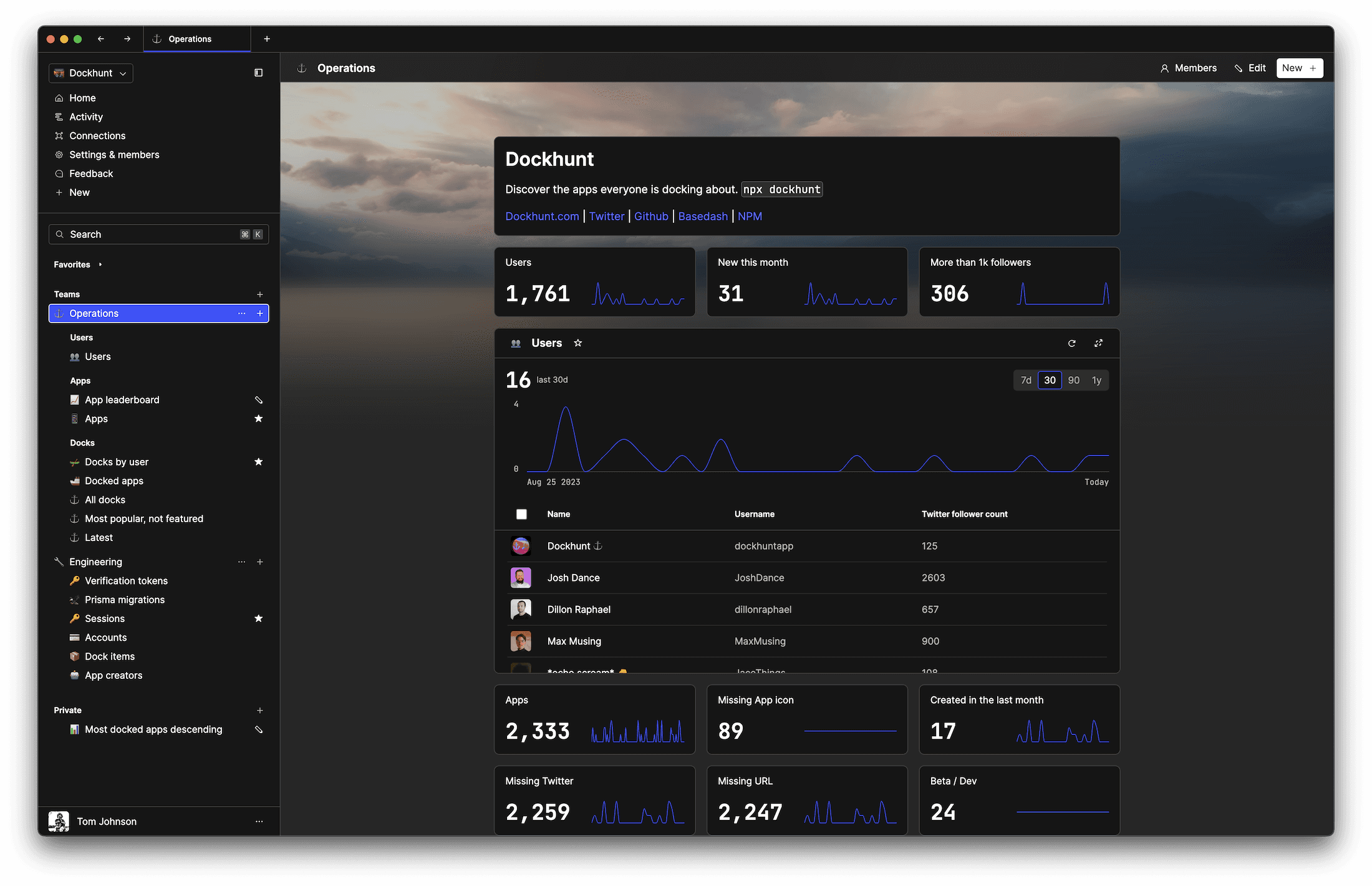SOC II
Type 2 Certified

### Features

Proudly carbon-neutral.SOC II
Type 2 Certified

### Features

Proudly carbon-neutral.SOC II
Type 2 Certified